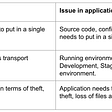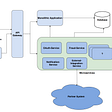# First Missing Positive Number

Given an unsorted integer array `nums`, return the smallest missing positive integer.

You must implement an algorithm that runs in `O(n)` time and uses constant extra space.

Example 1:

`Input: nums = [1,2,0]Output: 3`

Example 2:

`Input: nums = [3,4,-1,1]Output: 2`

Example 3:

`Input: nums = [7,8,9,11,12]Output: 1`

Constraints:

• `1 <= nums.length <= 5 * 105`
• `-231 <= nums[i] <= 231 - 1`

Algorithm:

The basic idea is that we have an array with n cells (n is the length of the array). If an integer is missing it must be in the range `[1..n]`. This is the crucial observation we use to deduce the algorithm. This means that the range of possible answers is [1..n] if an integer is missing, and if an integer is not missing then the answer is `n+1`.

Let’s picture the only two possibilities:

1. there is no missing integer in the array
2. there is a missing integer in the array.

If there is no missing integer, this means that the array has all number from 1 to n. This must mean that the array is full. Why, because in the range [1..n] there are exactly n numbers, and if you place n numbers in an array of length n, the array is by definition full. (in this case solution is to return n+1 which is the first smallest integer).

Once you understand the first case above understanding the second is easy. If there is a missing integer (or more than one), the missing integer(s), let’s call it X, must be in the range 1..n. Why, because if the missing integer X is not in the range [1..n] that would imply that all integers [1..n] are in the array, which would mean that the array is full, leaving no space where to place X (since X is not in the range [1..n]).

Then the algorithm becomes:

1- Ignore all numbers <=0 and >n since they are outside the range of possible answers (which we proved was [1..n]). We do this by replacing them with the value 1.
2- For all other integers <n+1, mark their bucket (cell) to indicate the integer exists(change the existing positive to negative).
3- Find the first cell not marked, that is the first missing integer. If you did not find an unmarked cell, there was no missing integer, so return n+1.

Solution

Complexity

Runtime: O(N)

Space: O(1)

--

--

--

## More from Jaydeep

Full Stack Programmer, love to solve problem’s during free time.

Love podcasts or audiobooks? Learn on the go with our new app.

## Recommended from Medium## 5 most dangerous risks in software development every project manager should know## Efficient code logistics.## Kitchen Assist — Product Hunt Deliverable 2 Update## Magic Select & Friends## Swift 3 Enums and Binary Trees## The Steps from Your Monolithic Application to Became at Scale## Jaydeep

Full Stack Programmer, love to solve problem’s during free time.

## Preparing for your Campus Placements (IT)## College Series: Applying to Companies & Preparing for Interviews## MICROSOFT : SWE’22 INTERN Interview Experience.## Longest Substring Without Repeating Characters